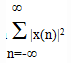Test: Discrete Time Signals

# Test: Discrete Time Signals

Test Description

## 10 Questions MCQ Test Digital Signal Processing | Test: Discrete Time Signals

Test: Discrete Time Signals for Electrical Engineering (EE) 2022 is part of Digital Signal Processing preparation. The Test: Discrete Time Signals questions and answers have been prepared according to the Electrical Engineering (EE) exam syllabus.The Test: Discrete Time Signals MCQs are made for Electrical Engineering (EE) 2022 Exam. Find important definitions, questions, notes, meanings, examples, exercises, MCQs and online tests for Test: Discrete Time Signals below.
Solutions of Test: Discrete Time Signals questions in English are available as part of our Digital Signal Processing for Electrical Engineering (EE) & Test: Discrete Time Signals solutions in Hindi for Digital Signal Processing course. Download more important topics, notes, lectures and mock test series for Electrical Engineering (EE) Exam by signing up for free. Attempt Test: Discrete Time Signals | 10 questions in 10 minutes | Mock test for Electrical Engineering (EE) preparation | Free important questions MCQ to study Digital Signal Processing for Electrical Engineering (EE) Exam | Download free PDF with solutions
 1 Crore+ students have signed up on EduRev. Have you?
Test: Discrete Time Signals - Question 1

### If x(n) is a discrete-time signal, then the value of x(n) at non integer value of ‘n’ is:

Detailed Solution for Test: Discrete Time Signals - Question 1

Explanation: For a discrete time signal, the value of x(n) exists only at integral values of n. So, for a non- integer value of ‘n’ the value of x(n) does not exist.

Test: Discrete Time Signals - Question 2

### The discrete time function defined as u(n)=n for n≥0;=0 for n<0 is an:

Detailed Solution for Test: Discrete Time Signals - Question 2

Explanation: When we plot the graph for the given function, we get a straight line passing through origin with a unit positive slope. So, the function is called as unit ramp signal.

Test: Discrete Time Signals - Question 3

### The phase function of a discrete time signal x(n)=an, where a=r.ejθ is:

Detailed Solution for Test: Discrete Time Signals - Question 3

Explanation: Given x(n)=an=(r.e)n =rn.ejnθ
=>x(n)=rn.(cosnθ+jsinnθ)
Phase function is tan-1(cosnθ/sinnθ)=tan-1(tan nθ)=nθ.

Test: Discrete Time Signals - Question 4

The signal given by the equationis known as:

Detailed Solution for Test: Discrete Time Signals - Question 4

Explanation: We have used the magnitude-squared values of x(n), so that our definition applies to complex-valued as well as real-valued signals. If the energy of the signal is finite i.e., 0<E<∞ then the given signal is known as Energy signal.

Test: Discrete Time Signals - Question 5

x(n)*δ(n-k)=?

Detailed Solution for Test: Discrete Time Signals - Question 5

Explanation: The given signal is defined only when n=k by the definition of delta function. So, x(n)*δ(n-k)= x(k)*δ(n-k).

Test: Discrete Time Signals - Question 6

A real valued signal x(n) is called as anti-symmetric if:

Detailed Solution for Test: Discrete Time Signals - Question 6

Explanation: According to the definition of anti-symmetric signal, the signal x(n) should be symmetric over origin. So, for the signal x(n) to be symmetric, it should satisfy the condition x(n)=-x(-n).

Test: Discrete Time Signals - Question 7

The odd part of a signal x(t) is:

Detailed Solution for Test: Discrete Time Signals - Question 7

Explanation: Let x(t)=xe(t)+xo(t)
=>x(-t)=xe(-t)-xo(-t)
By subtracting the above two equations, we get
xo(t)=(1/2)*(x(t)-x(-t)).

Test: Discrete Time Signals - Question 8

Time scaling operation is also known as:

Detailed Solution for Test: Discrete Time Signals - Question 8

Explanation: If the signal x(n) was originally obtained by sampling a signal xa(t), then x(n)=xa(nT). Now, y(n)=x(2n)(say)=xa(2nT). Hence the time scaling operation is equivalent to changing the sampling rate from 1/T to 1/2T, that is to decrease the rate by a factor of 2. So, time scaling is also called as down-sampling.

Test: Discrete Time Signals - Question 9

What is the condition for a signal x(n)=Brn where r=eαT to be called as an decaying exponential signal?

Detailed Solution for Test: Discrete Time Signals - Question 9

Explanation: When the value of ‘r’ lies between 0 and 1 then the value of x(n) goes on decreasing exponentially with increase in value of ‘n’. So, the signal is called as decaying exponential signal.

Test: Discrete Time Signals - Question 10

The function given by the equation x(n)=1, for n=0;=0, for n≠0 is a:

Detailed Solution for Test: Discrete Time Signals - Question 10

Explanation: According to the definition of the impulse function, it is defined only at n=0 and is not defined elsewhere which is as per the signal given.

## Digital Signal Processing

3 videos|50 docs|54 tests
 Use Code STAYHOME200 and get INR 200 additional OFF Use Coupon Code
Information about Test: Discrete Time Signals Page
In this test you can find the Exam questions for Test: Discrete Time Signals solved & explained in the simplest way possible. Besides giving Questions and answers for Test: Discrete Time Signals, EduRev gives you an ample number of Online tests for practice

## Digital Signal Processing

3 videos|50 docs|54 tests